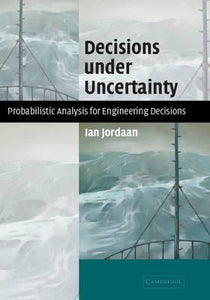# Decisions under Uncertainty : Probabilistic Analysis for Engineering Decisions by Jordaan, Ian

• R 400.00
Unit price per
Tax included.

ISBN: 9780521782777

CAMBRIDGE UNIVERSITY PRESS, 5 September 2011

Hardback 690 pages

To better understand the core concepts of probability and to see how they affect real-world decisions about design and system performance, engineers and scientists might want to ask themselves the following questions: what exactly is meant by probability? What is the precise definition of the 100-year load and how is it calculated? What is an 'extremal' probability distribution? What is the Bayesian approach? How is utility defined? How do games fit into probability theory? What is entropy? How do I apply these ideas in risk analysis? Starting from the most basic assumptions, this 2005 book develops a coherent theory of probability and broadens it into applications in decision theory, design, and risk analysis. This book is written for engineers and scientists interested in probability and risk. It can be used by undergraduates, graduate students, or practicing engineers.